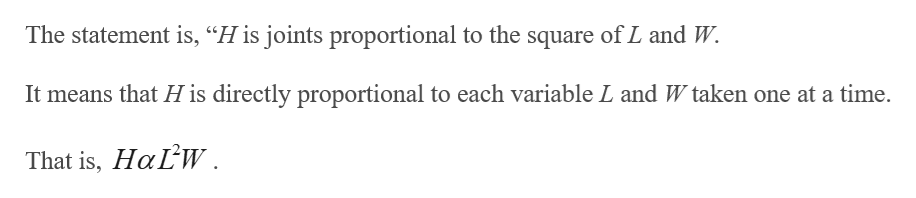a.) Express the statement as an equation. (Use k as the constant of proportionality.)H is jointly proportional to the squares of L and W. b.)Use the given information to find the constant of proportionality.If L = 3 and W = 1/2, then H = 45.

Question

a.) Express the statement as an equation. (Use k as the constant of proportionality.)

H is jointly proportional to the squares of L and W.

b.)Use the given information to find the constant of proportionality.
If
L = 3 and W = 1/2, then H = 45.
Step 1

a) Express the statement...help_outlineImage TranscriptioncloseThe statement is, "H is joints proportional to the square of L and W. It means that H is directly proportional to each variable L and W taken one at a time. That is, HaLW. fullscreen

Want to see the full answer?

See Solution

Want to see this answer and more?

Our solutions are written by experts, many with advanced degrees, and available 24/7

See Solution
Tagged in

Other